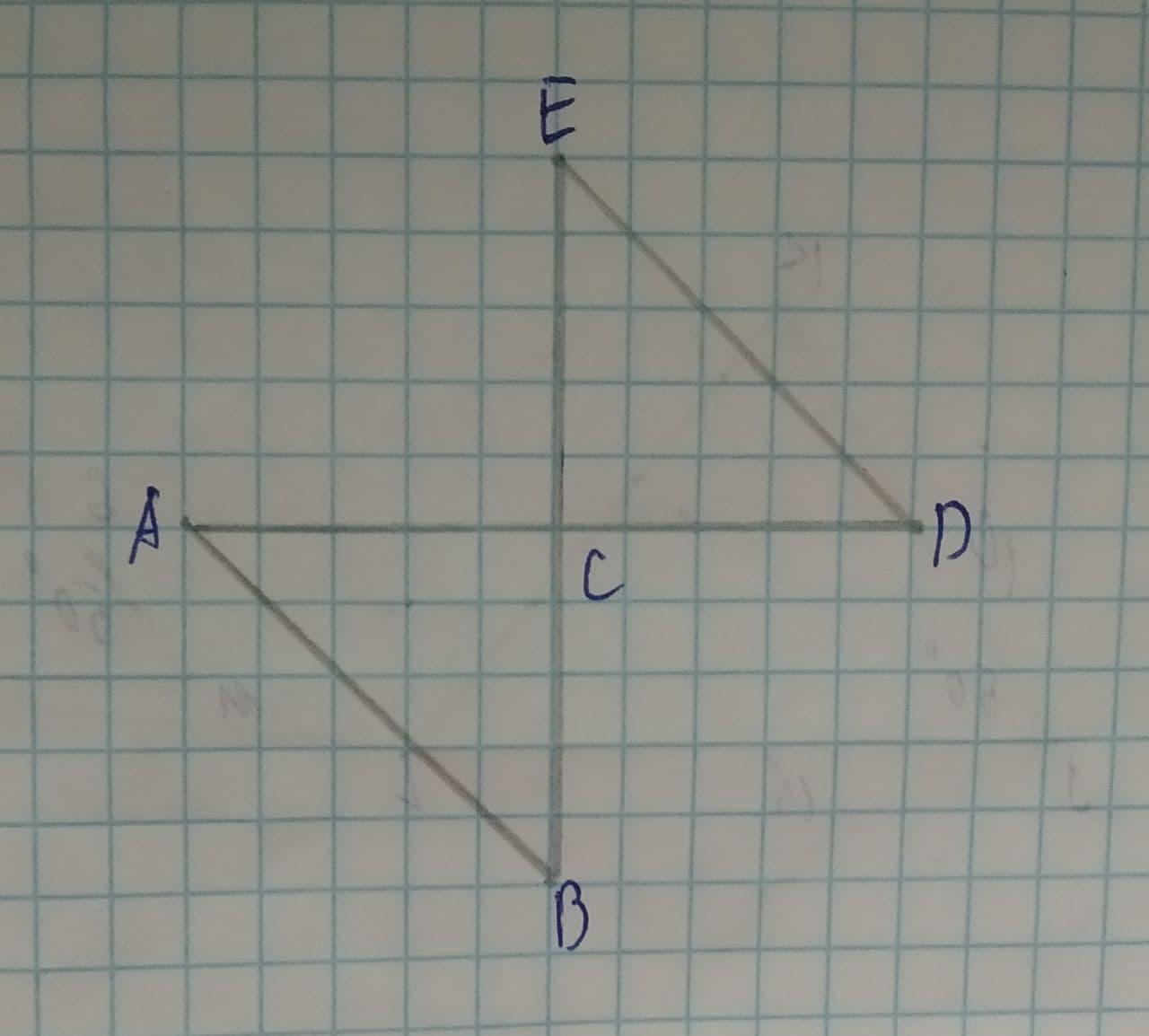# Write a proof for the following \overline{AB}\cong \overline{DE} and C is the midpoint of both \overline{AB}\ and\ \overline{BE}lwfrgin 2021-08-09 Answered
Write a proof for the following:
Given $\stackrel{―}{AB}\stackrel{\sim }{=}\stackrel{―}{DE}$ and C is the midpoint of both .
Prove: $\mathrm{△}ABC\stackrel{\sim }{=}\mathrm{△}DEC$.You can still ask an expert for help

• Questions are typically answered in as fast as 30 minutes

Solve your problem for the price of one coffee

• Math expert for every subject
• Pay only if we can solve itcurwyrm
Step 1
$AB\stackrel{\sim }{=}DE$ and C is the mid point of both AD and BE.
Now
AC=DC...(1) ($\because$ C is mid point of AD)
CE=BC...(2) ($\because$ C is the mid point of BE)
$\mathrm{\angle }ACB=\mathrm{\angle }BCE$...(3) ($\because$ vertically opposite $\mathrm{\angle }s$)
Also $AB\stackrel{\sim }{=}DE$...(4) (Given)
Step 2
Therefore from (1), (2) and (4)
$\mathrm{△}ABC\stackrel{\sim }{=}\mathrm{△}DEC$ (by SSS similarity)
Also from (1), (2) and (4)
$\mathrm{△}ABC\stackrel{\sim }{=}\mathrm{△}DEC$ (by SAS similarity)